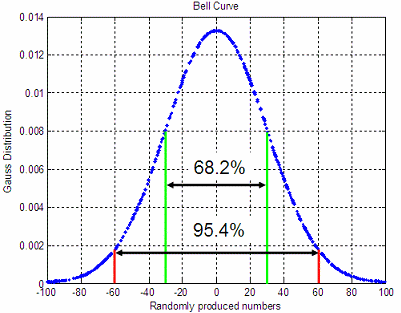# Gaussian distribution – how to plot it in Matlab

 In statistics and probability theory, the Gaussian distribution is a continuous distribution that gives a good description of data that cluster around a mean. The graph or plot of the associated probability density has a peak at the mean, and is known as the Gaussian function or bell curve. The Probability Density Function (PDF) in this case can be defined as:whereThe formula above can me coded in Matlab easily, like this:

function f = gauss_distribution(x, mu, s)
p1 = -.5 * ((x - mu)/s) .^ 2;
p2 = (s * sqrt(2*pi));
f = exp(p1) ./ p2;

Now, let’s use it in an example.

We produce 500 random numbers between -100 and 100, with mean m = 0 and standard deviation s = 30. The code is:

a = -100; b = 100;
x = a + (b-a) * rand(1, 500);
m = (a + b)/2;
s = 30;

Then, we plot this information using our bell curve:

f = gauss_distribution(x, m, s);
plot(x,f,
'.')
grid
on
title('Bell Curve')
xlabel(
'Randomly produced numbers')
ylabel(
'Gauss Distribution')

The produced shape is:An important property of this bell-shaped curve is that the values less than one standard deviation from the mean (between green lines below) represent approximately 68.2% of the area under the curve, while two standard deviations from the mean (between red lines below) take about 95.4%, and three standard deviations account for about 99.7% of the area.From 'Gaussian distribution' to home

From 'Gaussian distribution' to Matlab 2D plots

Probability and Statistics

 Statistics: Normal Distribution Top